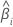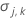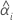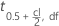# Methods and formulas for the shelf life for the specified percent method for Stability Study for fixed batches

## Shelf life for a model with a fixed batch factor and only a lower specification limit

To simplify the calculation of the shelf life, consider which model you fit to the data.

### The model with time, batch, and the time*batch interaction

When the batch effect and the batch*time interaction are in the model, the fit for the ith batch at time xij uses the model that follows:

To find the shelf life, set the equation that follows equal to the lower specification limit and solve for time (x).

where

I = the total number of batch levels

n = the total number of response values

X = the design matrix for the model

To calculate a meaningful shelf life, Minitab evaluates three conditions. First, Minitab determines whether the mean response is statistically greater than the lower specification limit at time = 0.

Second, Minitab determines whether the mean response declines at a statistically significant rate over time.

Third, Minitab determines whether the square root portion of the quadratic equation has a real number solution.

where

If all three conditions are true then Minitab calculates the shelf life. To calculate the shelf life, use the quadratic equation.

### The model with time and batch

When the batch*time interaction is not in the model, the slopes are the same for every batch. The fit for the ith batch at time xij uses the model that follows:

To find the shelf life, set the equation that follows equal to the lower specification limit and solve for time (x).

where

I = the total number of batch levels

n = the total number of response values

X = the design matrix for the model

To calculate a meaningful shelf life, Minitab evaluates three conditions. First, Minitab determines whether the mean response is statistically greater than the lower specification limit at time = 0.

Second, Minitab determines whether the mean response declines at a statistically significant rate over time.

Third, Minitab determines whether the square root portion of the quadratic equation has a real number solution.

where

If all three conditions are true then Minitab calculates the shelf life. To calculate the shelf life, use the quadratic equation.

### The model with time

When only time is in the model, the slopes and intercepts are the same for every batch. The fit at time xij uses the model that follows:

To find the shelf life, set the equation that follows equal to the lower specification limit and solve for time (x).

where

I = the total number of batch levels

n = the total number of response values

X = the design matrix for the model

To calculate a meaningful shelf life, Minitab evaluates three conditions. First, Minitab determines whether the mean response is greater than the lower specification limit at time = 0.

Second, Minitab determines whether the mean response declines over time.

Third, Minitab determines whether the square root portion of the quadratic equation has a real number solution.

where

If all three conditions are true then Minitab calculates the shelf life. To calculate the shelf life, use the quadratic equation.

### Notation

TermDescriptionthe slope for the ith batch
Zthe value of the inverse cumulative probability function from the standard normal distribution with the given probabilitythe variance of the estimated parameter vectorthe intercept for the ith batch
S2the mean square error
Lthe lower specification limit
Xthe design matrix
ian index to show the batch that the shelf life estimate is for
lthe number of levels in the batch factor
nthe total number of response values

## Shelf life for a model with a fixed batch factor and only an upper specification limit

To simplify the calculation of the shelf life, consider which model you fit to the data.

### The model with time, batch, and the time*batch interaction

When the batch effect and the batch*time interaction are in the model, the fit for the ith batch at time xij uses the model that follows:

To find the shelf life, set the equation that follows equal to the upper specification limit and solve for time (x).

where

I = the total number of batch levels

n = the total number of response values

X = the design matrix for the model

To calculate a meaningful shelf life, Minitab evaluates three conditions. First, Minitab determines whether the mean response is statistically less than the upper specification limit at time = 0.

Second, Minitab determines whether the mean response increases at a statistically significant rate over time.

Third, Minitab determines whether the square root portion of the quadratic equation has a real number solution.

where

If all three conditions are true then Minitab calculates the shelf life. To calculate the shelf life, use the quadratic equation.

### The model with time and batch

When the batch*time interaction is not in the model, the slopes are the same for every batch. The fit for the ith batch at time xij uses the model that follows:

To find the shelf life, set the equation that follows equal to the upper specification limit and solve for time (x).

where

I = the total number of batch levels

n = the total number of response values

X = the design matrix for the model

To calculate a meaningful shelf life, Minitab evaluates three conditions. First, Minitab determines whether the mean response is statistically less than the upper specification limit at time = 0.

Second, Minitab determines whether the mean response increases at a statistically significant rate over time.

Third, Minitab determines whether the square root portion of the quadratic equation has a real number solution.

where

If all three conditions are true then Minitab calculates the shelf life. To calculate the shelf life, use the quadratic equation.

### The model with time

When only time is in the model, the slopes and intercepts are the same for every batch. The fit at time xij uses the model that follows:

To find the shelf life, set the equation that follows equal to the upper specification limit and solve for time (x).

where

I = the total number of batch levels

n = the total number of response values

X = the design matrix for the model

To calculate a meaningful shelf life, Minitab evaluates three conditions. First, Minitab determines whether the mean response is less than the upper specification limit at time = 0.

Second, Minitab determines whether the mean response increases over time.

Third, Minitab determines whether the square root portion of the quadratic equation has a real number solution.

where

If all three conditions are true then Minitab calculates the shelf life. To calculate the shelf life, use the quadratic equation.

### Notation

TermDescriptionthe slope for the ith batch
Zthe value of the inverse cumulative probability function from the standard normal distribution with the given probabilitythe variance of the estimated parameter vectorthe intercept for the ith batch
S2the mean square error
Uthe upper specification limit
Xthe design matrix
ian index to show the batch that the shelf life estimate is for
lthe number of levels in the batch factor
nthe total number of response values

## Both limits

To simplify the calculation of the condition for how and when Minitab calculates the shelf life, consider which model you fit to the data.

### The model with time, batch, and the time*batch interaction

Minitab evaluates two conditions to determine whether a meaningful estimate of the shelf life exists. First, Minitab determines whether the mean response is statistically within the specification limits.

where

TermDescription
Ithe total number of batch levels
nthe total number of response values
Xthe design matrix for the model

Second, Minitab determines whether the mean response changes at a statistically significant rate over time.

If a meaningful estimate exists, then Minitab determines whether the mean response increases or decreases over time. If the second conditions is false, then one of the conditions that follows is true.

If the response decreases with time, the Minitab calculates the shelf life relative to the lower specification limit. This formula gives the condition when the response decreases:

If the response increases with time, then Minitab calculates the shelf life relative to the upper specification limit. This formula gives the condition when the response increases:

If the mean response decreases over time, then Minitab calculates the shelf life relative to the lower specification limit. Otherwise, Minitab calculates the shelf life relative to the upper specification limit.

For details on the calculation of the shelf life for each case, go to the corresponding section about the shelf life for that specification limit.

### The model with time and batch

Minitab evaluates two conditions to determine whether a meaningful estimate of the shelf life exists. First, Minitab determines whether the mean response is statistically within the specification limits.

where

TermDescription
Ithe total number of batch levels
nthe total number of response values
Xthe design matrix for the model

Second, Minitab determines whether the mean response changes at a statistically significant rate over time.

If a meaningful estimate exists, then Minitab determines whether to estimate the shelf life relative to the upper specification limit or the lower specification limit.

If the response decreases with time, then Minitab calculates the shelf life relative to the lower specification limit. This formula gives the condition when the response decreases:
If the response increases with time, then Minitab calculates the shelf life relative to the upper specification limit. This formula gives the condition when the response increases:

For details on the calculation of the shelf life for each case, go to the corresponding section about the shelf life for that specification limit.

### The model with time

Minitab evaluates two conditions to determine whether a meaningful estimate of the shelf life exists. First, Minitab determines whether the mean response is statistically within the specification limits.

where

TermDescription
Ithe total number of batch levels
nthe total number of response values
Xthe design matrix for the model

Second, Minitab determines whether the mean response changes at a statistically significant rate over time.

If a meaningful estimate exists, then Minitab determines whether to estimate the shelf life relative to the upper specification limit or the lower specification limit.

If the response decreases with time, then Minitab calculates the shelf life relative to the lower specification limit. This formula gives the condition when the response decreases:
If the response increases with time, then Minitab calculates the shelf life relative to the upper specification limit. This formula gives the condition when the response increases:

For details on the calculation of the shelf life for each case, go to the corresponding section about the shelf life for that specification limit.

### Notation

TermDescriptionThe slope for the ith batch
Ithe number of levels in the batch factor
nthe number of rows in the datathe value of the inverse cumulative distribution at 0.5+cl/2 from the t distribution with df degrees of freedom
By using this site you agree to the use of cookies for analytics and personalized content.  Read our policy